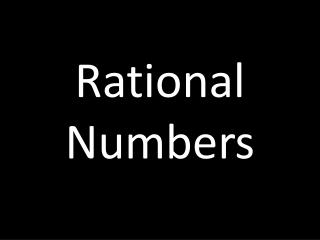DownloadDownload PresentationRational Numbers

# Rational Numbers

Télécharger la présentation## Rational Numbers

- - - - - - - - - - - - - - - - - - - - - - - - - - - E N D - - - - - - - - - - - - - - - - - - - - - - - - - - -
##### Presentation Transcript

1. Rational Numbers

2. Some Definitions • Rational Number: Any number that can be converted into a fraction ( Examples: ¼, 3, 4.25, 0). • Fraction: A part of a whole ( ¼ , ¾). • Mixed Number: A whole number and a fraction (1 ¼ ) • Terminating Decimal: A decimal number that stops (3.75, 4.3, 8.3245). • Repeating Decimal: A decimal number that has a repeating pattern (3.757575… , 2.345345… )

3. Adding Decimal Numbers • To add decimal numbers, line up the addends by the decimal place and add. • Make sure the place values line up properly.

4. Complete #1-5

5. Applying Integer Rules • We can apply the same rules for integers to rational decimal numbers. • Rule 1: If the signs are the same, add and keep the sign the same. • Rule 2: If the signs are different, subtract and keep the sign of the larger number.

6. Complete #6-10

7. Adding Fractions and Mixed Numbers • When you add fractions, you must first find a common denominator. • We find a common denominator by finding the least common multiple for the denominators of our addends. • Make sure your answer is always in lowest terms.

8. Complete #11-15

9. Applying Integer Rules • We can also apply our integer rules to fractions and mixed numbers. • If we need to borrow, take one away from the whole number and add the appropriate amount to the numerator.

10. Complete #16-20

11. Subtracting Rational Numbers

12. Use your subtraction rules: • Change subtraction to addition and find the opposite of the second number. • Go back to addition rules.

13. Multiplying Rational Numbers

14. Multiplying Decimals • Line up the numbers justified right. • Multiply the factors. • Count the decimal places in each factor and add. • Move the decimal place that many times to the left.

15. Multiplying Fractions • Change mixed numbers to improper fractions. • Cross cancel and multiply OR • Multiply and reduce

16. Dividing Rational Numbers

17. Dividing Decimals • Set it up. (First in) • Move the decimal on the outside. • Move the decimal on the inside the same number of times. • Rewrite and divide.

18. Dividing Fractions • Change mixed numbers to improper fractions. • KFC